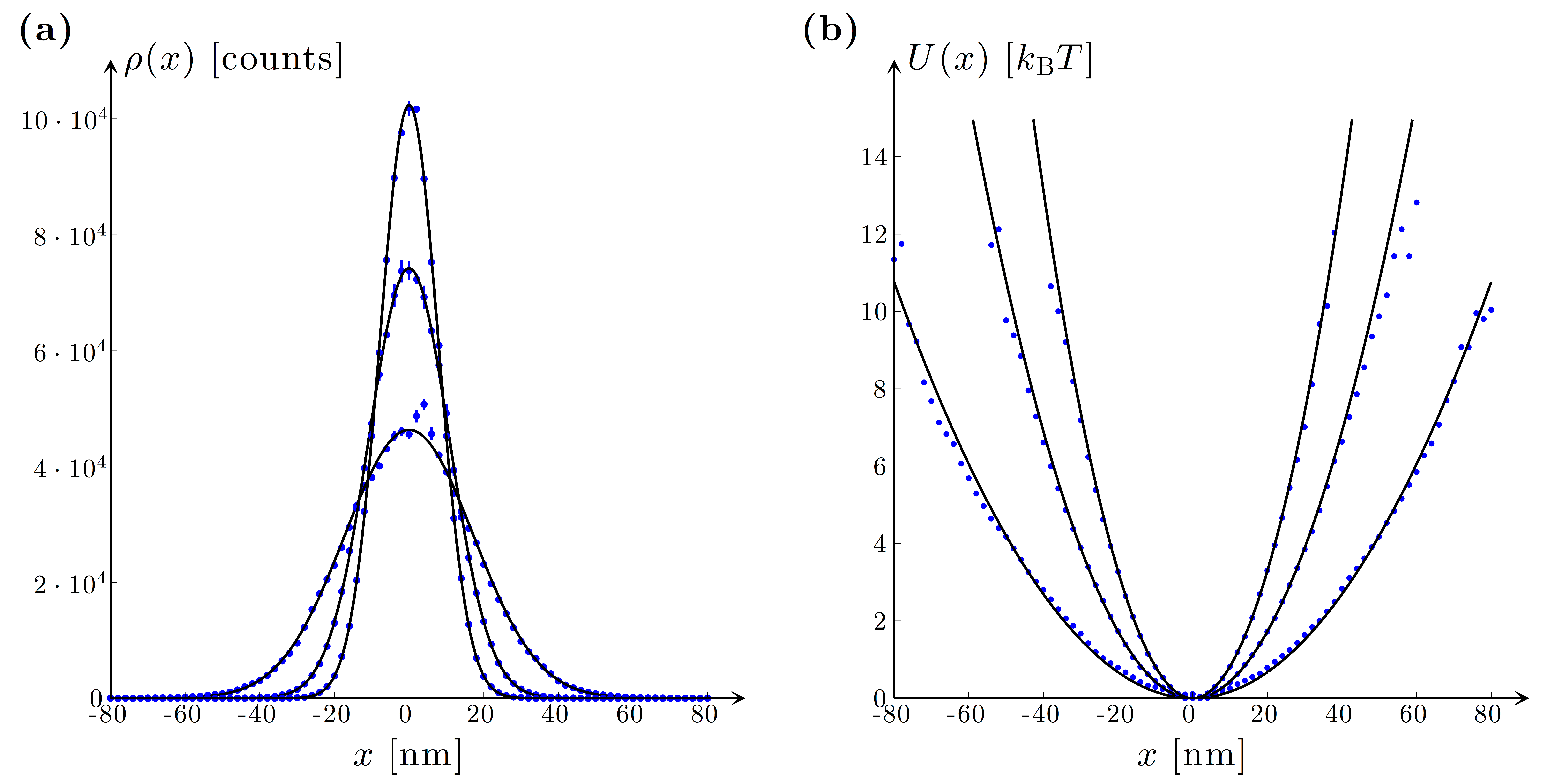# Figure 9.10 — Potential and equipartition analysisFigure 9.10 — Potential and equipartition analysis. (a) Position histograms and (b) potentials corresponding to an optically trapped polystyrene sphere (a = 1.03 μm). The symbols represent the potential method analysis of three series of experimental data corresponding to 2.3, 6.0 and 10.8 mW laser power (λ0 = 1064 nm) at the sample. The solid lines correspond to the position distribution and the potential of an optical trap with the stiffness obtained from the equipartition analysis [Eq. (9.28)] of the experimental data (κx(ex) = 13.9 ± 0.3, 35.7 ±0.7 and 68.0 ± 0.3 fN/nm). These data demonstrate that the trap’s potential is harmonic up to 10 kBT at least.